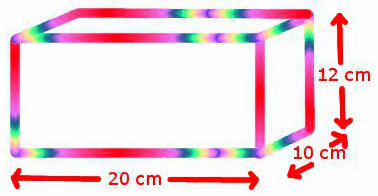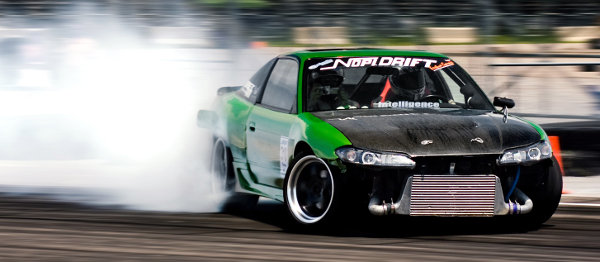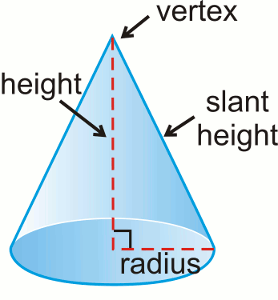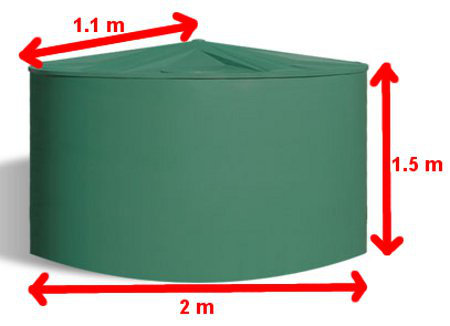# Surface Area Of Prisms And Pyramids

To calculate the Surface Area (SA) of a 3-dimensional solid, we add up the areas of all the faces.

Examples where surface area is calculated include:

• the cardboard of a box
• the metal in a can
• the plastic walls of a water tank
• the enamel of a saucepan

## Example One - Amount of Cardboard in a BoxThis cardboard box has the shape of a rectangular prism with a length of 20cm, a width of 10 cm and a height of 12 cm. If it has a lid, how much cardboard is needed to make the box?

There are 3 differently-sized rectangles with 3 different pairs of measurements.

 Area of 1st rectangle = 20 × 10 = 200 cm2 Area of 2nd rectangle = 20 × 12 = 240 cm2 Area of 3rd rectangle = 12 × 10 = 120 cm2 560 cm2

Surface Area
= 560 × 2
= 1120 cm2

## Example Two - Loss of Tyre Rubber When DriftingDrifting is an expensive and unhealthy past time. A thin layer of tyre rubber abrades, burns due to the friction of the tyre spinning, and then the tyre rubber particulates go into air to be inhaled. One tyre has a diameter of 50 cm and a tread width of 20 cm. What is area of tyre rubber that comes in contact with the road in one rotation of one tyre? (Use π = 3.14.)

The contact area is a rectangle with a length equal to the circumference of the tyre and a width of 20 cm.

Circumference of circle
= π × D
= 3.14 × 50
= 157 cm

Area of rectangle
= Circumference × Width
157 × 20
= 3140 cm2

## Did You Know That...?

Situations where surface area matters include:

• After a bath, drying with a fluffy towel is more effective than with a flat fabric because the extra filaments on the towel provide a greater area for contact between the water molecules and the towel and therefore increases absorption.
• When we wash our dirty clothes, the detergent acts in two ways. Firstly, detergent separates the dirt lumps into smaller particles. Secondly, detergent contains chemicals that attach to the dirt particle at one end and to the water particle at the other end, and this helps to wash the dirt down the drain.
• Bile, a digestive chemical produced by the human gall bladder, acts like a detergent to separate and disperse fat particles of food so that there is a greater contact area between the fat particles and the enzymes which breaks them down.
• In chemistry, we often grind chemicals into a fine powder before reacting them with other chemicals. The reason is that finely-ground particles have a greater surface area than larger lumps.
• Glues and welds work best when there is a greater contact area between two surfaces. Before gluing and welding, we clean the surfaces to remove any bumpy bits to make the surfaces smoother.

## Example Three - Painting a Square-Based PyramidThe Great Pyramid of Giza in Egypt was originally coated with a protective layer of white limestone. Its square base has a side of 230 metres. The sloped height is 300 metres. Work out the area of this coating.

 Area of one triangle = (B × H) = (230 × 300) = 34 500 m2 2 2

Area of 4 triangles = 34 500 × 4 = 138 000 m2

A CONE is a circle-based pyramid.

Area of Cone = π × Radius × Slant height## Example of Plastic Water TankThis water tank is composed of a cylinder as the bottom and a cone as the top. The diameter is 2 metres. The height of the cylinder is 1.5 metres. The sloped length (slant height) of the cone top from its peak to the edge is 1.1 metres. What area of plastic is used to make the tank? (Use π = 3.14.)

Round Base
Area of circle
= π r2
= 3.14 × 1 × 1
= 3.14 m2

Cylindrical Wall
Circumference of circle
= π × D
= 3.14 × 2
= 6.28 m

Area of rectangle
= Circumference × Height
= 6.28 × 1.5
= 9.42 m2

Open Cone Top
Surface Area of cone
= π × r × s
= 3.14 × 1 × 1.1
= 3.45 m2

Total Surface Area
SA = 3.14 + 6.28 + 3.45
= 12.87 m2

## Questions

Q1. Toblerone Chocolate is packaged in long boxes with the shape of a triangular prism. The end triangles are equilateral triangles with a base of 4 cm and a vertical height of 3.5 cm. The box length is 30 cm. What area of cardboard is used to make one box?

Q2. A can of Coke has these approximate dimensions - a diameter of 6.4 cm and a height of 13 cm. What area of metal makes one can? (Use Pi = 3.14. Remember it has a top and a bottom.)